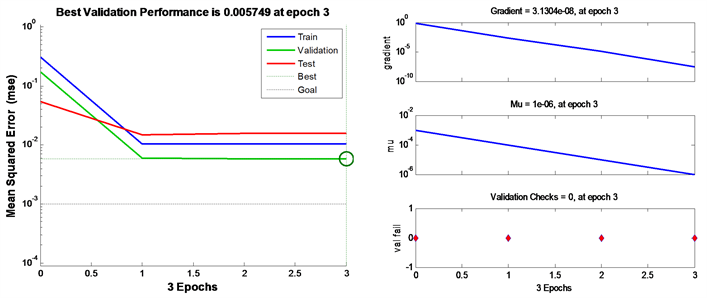﻿ 基于BP神经网络的全变差模型参数选择

# 基于BP神经网络的全变差模型参数选择Parameter Selection of Total Variation Model Based on BP Neural Network

Abstract: The energy functional minimization proposed by the variational theory has been widely used in image denoising. In the process of denoising, it is very important to select parameters reasonably. The versatility and operability of neural networks have more advantages than traditional algo-rithms. In this paper, the shortcomings of the regular variable parameter cannot be adaptively adjusted in the total variation denoising model. By constructing the BP neural network model, after a lot of learning and training, the relationship between the original image information and the regular term parameters is simulated, and the regular term obtained from the model is obtained. The parameters, combined with the Chambolle dual algorithm, form a whole, so that the improved algorithm has more accurate parameter selection and more effective denoising effect.

1. 引言

ROF模型中的能量泛函分为逼近项和正则项两部分，其中，逼近项用于去除噪声，保证去噪后的图像与原图像的相似性，而正则项则防止去噪过强，起到抑制噪声的作用，因此，需要选取适当的权重参数用于平衡这两项的关系，目前，国内外许多学者都已经提出了一些权重确定方法，具体有无偏预测风险估计器  (URPE)，局部方差估计  ，基于Morozov偏差原理  选取参数等方法。本文基于全变差正则化模型，利用Chambolle对偶投影算法   ，提出了一种参数可以随着迭代算法自适应地改变的新方法，可根据图像的先验信息，能够自适应的选取合适的参数，进而保证去噪后的图像能够保留更多的细节信息。

2. 模型的建立

2.1. ROF模型

ROF模型的基本思想是将图像处理问题看成一个能量系统，其中，不含噪声的图像能量相对比较小。当图像被噪声污染后，图像就变得不光滑，其能量也就相应的增大了很多，通过这种思想，就将图像去噪问题转化成了一个能量最小化问题，之后，再通过变分法进行求解。模型如下

$\underset{u\in BV\left(\Omega \right)}{\mathrm{min}}E\left(u\right)=J\left(u\right)+\frac{\text{1}}{2\lambda }{‖u-f‖}^{2}$ (1)

2.2. Chambolle去噪对偶模型

$\text{0}\in \partial J\left(u\right)+\lambda \left(u-f\right)$ (2)

$u\in \partial {J}^{*}\left(\left(u-f\right)/\lambda \right)$ (3)

${J}^{\ast }={\chi }_{K}\left(v\right)=\left\{\begin{array}{ll}0\hfill & v\in K\hfill \\ +\infty \hfill & 其他\hfill \end{array}$ (4)

$\frac{f}{\lambda }\in \frac{f-u}{\lambda }+\frac{1}{\lambda }\partial {J}^{*}\left(\frac{f-u}{\lambda }\right)$

3. 模型的求解

3.1. 投影算法

$\underset{\omega }{\mathrm{min}}E\left(\omega \right)=\frac{\text{1}}{\text{2}}{‖\omega -\lambda f‖}_{2}^{2}+\lambda {J}^{*}\left(\omega \right)$ (5)

${J}^{*}$ 由(5)式已给出，我们可以推导出 $\omega$ 一定是 $\lambda f$ 在凸集K上的正交投影，若 ${P}_{{K}_{\lambda }}$ 表示在集合K上的

$u=f-{P}_{{K}_{\lambda }}\left(f\right)$ (6)

$\underset{p}{\mathrm{min}}\left\{{‖\lambda divp-f‖}_{2}:p\in Y,{|{P}_{i,j}|}^{2}-1\le 0,\forall i,j=1,\cdots ,N\right\}$ (7)

$-{\left(\nabla \left(\lambda divp-f\right)\right)}_{i,j}+{\alpha }_{i,j}{p}_{i,j}=0$ (8)

${p}_{i,j}^{n+1}={p}_{i,j}^{n}+\tau \left({\left(\nabla \left(div{p}^{n}-\frac{f}{\lambda }\right)\right)}_{i,j}-|{\left(\nabla \left(div{p}^{n}-\frac{f}{\lambda }\right)\right)}_{i,j}|\cdot {p}_{i,j}^{n}\right)$ (9)

3.2. BP神经网络

BP神经网络在机构上类似于多层感知器，是一种多层前馈神经网络，在结构上具有三层或三层以上神经元，包括输入层、中间层、和输出层。上下层之间实现全连接，而每层神经元之间无链接。当一对学习样本提供给网络后，神经元的激活值从输入层经各中间层向输出层传播，在输出层的各神经元获得网络的输入相应。

BP网络学习规则

1) 初始化。给每个连接权值 ${w}_{i,j},{v}_{jt}$ 、阈值 ${\theta }_{j}$${\gamma }_{t}$ 赋予区间 $\left(-1,1\right)$ 内是随机值。

2) 随机选取一组输入和目标样本 ${P}_{k}=\left({a}_{1}^{k},{a}_{2}^{k},\cdots ,{a}_{n}^{k}\right),{T}_{k}=\left({S}_{1}^{k},{S}_{2}^{k},\cdots ,{S}_{n}^{k}\right)$ 提供给网络。

3) 用输入样本 ${P}_{k}=\left({a}_{1}^{k},{a}_{2}^{k},\cdots ,{a}_{n}^{k}\right)$ 连接权 ${w}_{i,j}$ 和阈值 ${\theta }_{j}$ 计算中间层各单元的输入 ${s}_{j}$ ，然后用 ${s}_{j}$ 通过传递函数计算中间各层单元的输出 ${b}_{j}$

${s}_{j}=\underset{i=1}{\overset{n}{\sum }}{w}_{ij}{a}_{i}-{\theta }_{j},\text{\hspace{0.17em}}\text{\hspace{0.17em}}{b}_{j}=f\left({s}_{j}\right),\text{\hspace{0.17em}}\text{\hspace{0.17em}}j=1,2,\cdots ,p$

4) 利用中间层的输出 ${b}_{j}$ 、连接权 ${v}_{jt}$ 和阈值 ${\gamma }_{t}$ 计算输出层各单元的输出 ${L}_{t}$ ，然后通过传递函数计算输出层各单元的响应 ${C}_{t}$

5) 利用网络目标向量 ${T}_{k}=\left({y}_{1},{y}_{2}\cdots ,{y}_{q}\right)$ ，网络的实际输出 ${C}_{t}$ ，计算输出层的各单元一般化误差 ${d}_{t}^{k}$ 。再利用一般化误差和各单元的输出来修正连接权和阈值。直到m个训练样本训练完毕。

4. 数值实验与分析Figure 1. Network training and iteration diagramFigure 2. Model fitting chartFigure 3. The first line is noise image and the second line is post-processing effect imageTable 1. Image quality index comparison

5. 结论

 Rudin, L.I., Osher, S. and Fatemi, E. (1992) Nonlinear Total Variation Based Noise Removal Algorithms. Physica D: Nonlinear Phenomena, 60, 259-268.
https://doi.org/10.1016/0167-2789(92)90242-F

 Meyer, Y. (2001) Os-cillating Patterns in Image Processing and Nonlinear Evolution Equations: The Fifteenth Dean Jacqueline B. Lewis Memorial Lectures. American Mathematical Society, Providence, 122.
https://doi.org/10.1090/ulect/022

 Buades, A. Coll, B. and Morel, J.M. (2005) Image Denoising by Non-Local Averaging. IEEE International Conference on Acoustics, Speech, and Signal Processing, Philadelphia, 23 March 2005.

 Bras, N.B., Bioucas-Dias, J. and Martins, R.C. (2012) An Alternating Direction Algorithm for Total Variation Reconstruction Distributed Parameters. IEEE Transactions on Image Processing, 21, 3004-3016.
https://doi.org/10.1109/TIP.2012.2188033

 Zhang, X., Burger, M. and Osher, S. (2011) A Unified Pri-mal-Dual Algorithm Framework Based on BregmanIteration. Journal of Scientific Computing, 46, 20-46.
https://doi.org/10.1007/s10915-010-9408-8

 Dong, Y., Hintermuller, M. and Rincon-Camacho, M. (2011) Automated Regularization Parameter Selection in Multi-Scale Total Variation Models for Image Restoration. Journal of Mathematical Imaging & Vision, 40, 82-104.
https://doi.org/10.1007/s10851-010-0248-9

 Clason, C., Jin, B. and Kunisch, K. (2010) A Duality-Based Splitting Method for L1-TV Image Restoration with Automatic Regularization Parameter Choice. SIAM Journal on Scientific Computing, 32, 1484-1505.
https://doi.org/10.1137/090768217

 Wen, Y.W. and Chan, R.H. (2012) Parameter Selection for To-tal-Variation-Based Image Restoration Using Discrepancy Principle. IEEE Transactions on Image Processing, 21, 1770-1781.
https://doi.org/10.1109/TIP.2011.2181401

 Chan, T., Marquina, A. and Mulet, P. (2000) High-Order Total Variation-Based Image Restoration. SIAM Journal on Scientific Computing, 22, 503-516.
https://doi.org/10.1137/S1064827598344169

 Chambolle, A. (2004) An Algorithm for Total Variation Min-imization and Applications. Journal of Mathematical Imaging and Vision, 20, 89-97.
https://doi.org/10.1023/B:JMIV.0000011321.19549.88

 Boyd, S., Parikh, N., Chu, E., et al. (2010) Distributed Optimization and Statistical Learning via the Alternating Direction Method of Multipliers. Foundations & Trends in Machine Learning, 3, 1-122.
https://doi.org/10.1561/2200000016

 Chambolle, A. (2005) Total Variation Minimization and a Class of Binary MRF Models. In: Proceedings of the 5th International Workshop on Energy Min-imization Methods in Computer Vision and Pattern Recognition, Springer, St. Augustine, 136-152.
https://doi.org/10.1007/11585978_10

Top